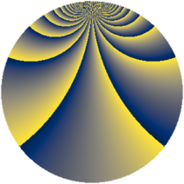# Properties

 Label 1183.2.bkLevel $1183$ Weight $2$ Character orbit 1183.bk Rep. character $\chi_{1183}(29,\cdot)$ Character field $\Q(\zeta_{39})$ Dimension $2208$ Sturm bound $242$

# Related objects

## Defining parameters

 Level: $$N$$ $$=$$ $$1183 = 7 \cdot 13^{2}$$ Weight: $$k$$ $$=$$ $$2$$ Character orbit: $$[\chi]$$ $$=$$ 1183.bk (of order $$39$$ and degree $$24$$) Character conductor: $$\operatorname{cond}(\chi)$$ $$=$$ $$169$$ Character field: $$\Q(\zeta_{39})$$ Sturm bound: $$242$$

## Dimensions

The following table gives the dimensions of various subspaces of $$M_{2}(1183, [\chi])$$.

Total New Old
Modular forms 2976 2208 768
Cusp forms 2880 2208 672
Eisenstein series 96 0 96

## Trace form

 $$2208q + 2q^{2} + 94q^{4} + 8q^{5} - 6q^{6} + 94q^{9} + O(q^{10})$$ $$2208q + 2q^{2} + 94q^{4} + 8q^{5} - 6q^{6} + 94q^{9} - 6q^{10} + 2q^{11} + 12q^{12} + 2q^{13} - 8q^{14} - 50q^{15} + 98q^{16} - 10q^{17} + 120q^{18} + 8q^{19} + 10q^{20} + 4q^{21} - 58q^{22} - 154q^{23} - 184q^{24} - 160q^{25} + 12q^{26} + 36q^{27} - 4q^{29} + 236q^{30} - 100q^{31} - 174q^{32} - 22q^{33} - 122q^{34} + 8q^{35} + 76q^{36} - 16q^{37} + 52q^{38} - 64q^{39} - 178q^{40} - 16q^{41} + 4q^{42} - 24q^{44} - 120q^{45} + 20q^{46} - 2q^{47} - 120q^{48} + 92q^{49} + 46q^{50} - 110q^{51} - 208q^{52} - 64q^{53} - 282q^{55} + 12q^{56} - 58q^{57} - 46q^{58} + 10q^{59} - 132q^{60} - 24q^{61} - 138q^{62} - 4q^{63} - 152q^{64} - 10q^{65} + 244q^{66} - 72q^{67} - 78q^{68} - 12q^{69} - 48q^{70} - 8q^{71} - 312q^{72} + 16q^{73} - 74q^{74} - 30q^{75} + 434q^{76} - 8q^{77} - 186q^{78} + 12q^{79} + 34q^{80} + 96q^{81} - 316q^{82} + 508q^{83} - 22q^{84} - 210q^{85} - 48q^{86} - 226q^{87} + 502q^{88} - 228q^{89} - 20q^{90} - 2q^{91} + 64q^{92} - 112q^{93} - 92q^{94} + 112q^{95} - 126q^{96} + 26q^{97} + 2q^{98} - 76q^{99} + O(q^{100})$$

## Decomposition of $$S_{2}^{\mathrm{new}}(1183, [\chi])$$ into newform subspaces

The newforms in this space have not yet been added to the LMFDB.

## Decomposition of $$S_{2}^{\mathrm{old}}(1183, [\chi])$$ into lower level spaces

$$S_{2}^{\mathrm{old}}(1183, [\chi]) \cong$$ $$S_{2}^{\mathrm{new}}(169, [\chi])$$$$^{\oplus 2}$$